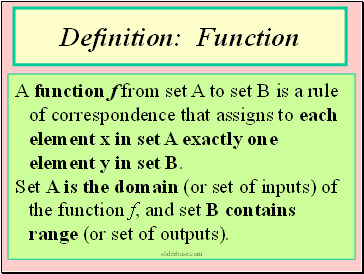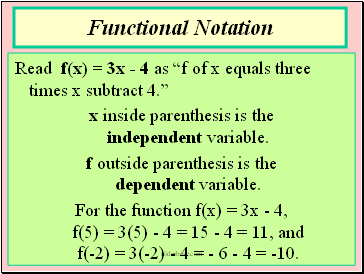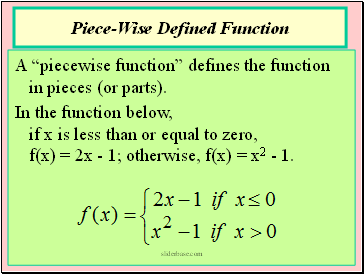# Functions and GraphsPage 1

#### WATCH ALL SLIDES

Slide 1PRECALCULUS I

Dr. Claude S. Moore Danville Community College

Functions and Graphs

Function, domain, independent variable

Graph, increasing/decreasing, even/odd

Slide 2## Definition: Function

A function f from set A to set B is a rule of correspondence that assigns to each element x in set A exactly one element y in set B.

Set A is the domain (or set of inputs) of the function f, and set B contains range (or set of outputs).

Slide 3## Characteristics of a Function

1. Each element in A (domain) must be matched with an element of B (range).

2. Each element in A is matched to not more than one element in B.

3. Some elements in B may not be matched with any element in A.

4. Two or more elements of A may be matched with the same element of B.

Slide 4## Functional Notation

Read f(x) = 3x - 4 as “f of x equals three times x subtract 4.”

x inside parenthesis is the independent variable.

f outside parenthesis is the dependent variable.

For the function f(x) = 3x - 4, f(5) = 3(5) - 4 = 15 - 4 = 11, and f(-2) = 3(-2) - 4 = - 6 - 4 = -10.

Slide 5## Piece-Wise Defined Function

A “piecewise function” defines the function in pieces (or parts).

In the function below, if x is less than or equal to zero, f(x) = 2x - 1; otherwise, f(x) = x2 - 1.

Slide 6A function f from set A to set B is a rule of correspondence that assigns to each element x in set A exactly one element y in set B.

Set A is the domain (or set of inputs) of the function f, and set B contains range (or set of outputs).

Definition: Function

Slide 7## Piece-Wise Defined Function

A “piecewise function” defines the function in pieces (or parts).

In the function below, if x is less than or equal to zero, f(x) = 2x - 1; otherwise, f(x) = x2 - 1.

Slide 8## Domain of a Function

Generally, the domain is implied to be the set of all real numbers that yield a real number functional value (in the range).

Some restrictions to domain:

1. Denominator cannot equal zero (0).

2. Radicand must be greater than or equal to zero (0).

3. Practical problems may limit domain.

Slide 9Go to page:
1  2